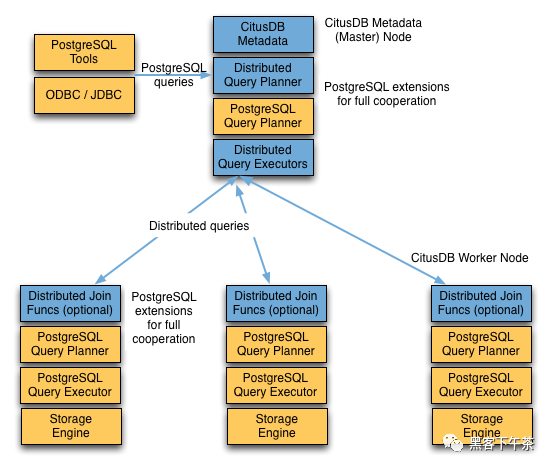# Citus 分布式 PostgreSQL 集群 – SQL Reference(查询处理)`Citus` 的查询处理管道涉及两个组件：

• 分布式查询计划器和执行器
• PostgreSQL 计划器和执行器

## 分布式查询计划器

`Citus` 的分布式查询计划器接收 `SQL` 查询并规划它以进行分布式执行。

## 分布式查询执行器

`Citus` 的分布式执行器运行分布式查询计划并处理故障。 执行器非常适合快速响应涉及过滤器、聚合和共置连接的查询，以及运行具有完整 `SQL` 覆盖的单租户查询。它根据需要为每个分片打开一个与 `woker` 的连接，并将所有片段查询发送给他们。 然后它从每个片段查询中获取结果，合并它们，并将最终结果返回给用户。

### 子查询/CTE Push-Pull 执行

``````SELECT page_id, count(distinct host_ip)
FROM page_views
WHERE page_id IN (
SELECT page_id
FROM page_views
GROUP BY page_id
ORDER BY count(*) DESC
LIMIT 20
)
GROUP BY page_id;
``````

``````GroupAggregate  (cost=0.00..0.00 rows=0 width=0)
Group Key: remote_scan.page_id
->  Sort  (cost=0.00..0.00 rows=0 width=0)
Sort Key: remote_scan.page_id
->  Custom Scan (Citus Adaptive)  (cost=0.00..0.00 rows=0 width=0)
->  Distributed Subplan 6_1
->  Limit  (cost=0.00..0.00 rows=0 width=0)
->  Sort  (cost=0.00..0.00 rows=0 width=0)
Sort Key: COALESCE((pg_catalog.sum((COALESCE((pg_catalog.sum(remote_scan.worker_column_2))::bigint, '0'::bigint))))::bigint, '0'::bigint) DESC
->  HashAggregate  (cost=0.00..0.00 rows=0 width=0)
Group Key: remote_scan.page_id
->  Custom Scan (Citus Adaptive)  (cost=0.00..0.00 rows=0 width=0)
Node: host=localhost port=9701 dbname=postgres
->  HashAggregate  (cost=54.70..56.70 rows=200 width=12)
Group Key: page_id
->  Seq Scan on page_views_102008 page_views  (cost=0.00..43.47 rows=2247 width=4)
Node: host=localhost port=9701 dbname=postgres
->  HashAggregate  (cost=84.50..86.75 rows=225 width=36)
Group Key: page_views.page_id, page_views.host_ip
->  Hash Join  (cost=17.00..78.88 rows=1124 width=36)
Hash Cond: (page_views.page_id = intermediate_result.page_id)
->  Seq Scan on page_views_102008 page_views  (cost=0.00..43.47 rows=2247 width=36)
->  Hash  (cost=14.50..14.50 rows=200 width=4)
->  HashAggregate  (cost=12.50..14.50 rows=200 width=4)
Group Key: intermediate_result.page_id
->  Function Scan on read_intermediate_result intermediate_result  (cost=0.00..10.00 rows=1000 width=4)
``````

``````GroupAggregate  (cost=0.00..0.00 rows=0 width=0)
Group Key: remote_scan.page_id
->  Sort  (cost=0.00..0.00 rows=0 width=0)
Sort Key: remote_scan.page_id
``````

``````    ->  Custom Scan (Citus Adaptive)  (cost=0.00..0.00 rows=0 width=0)
->  Distributed Subplan 6_1
.
``````

``````        ->  Limit  (cost=0.00..0.00 rows=0 width=0)
->  Sort  (cost=0.00..0.00 rows=0 width=0)
Sort Key: COALESCE((pg_catalog.sum((COALESCE((pg_catalog.sum(remote_scan.worker_column_2))::bigint, '0'::bigint))))::bigint, '0'::bigint) DESC
->  HashAggregate  (cost=0.00..0.00 rows=0 width=0)
Group Key: remote_scan.page_id
->  Custom Scan (Citus Adaptive)  (cost=0.00..0.00 rows=0 width=0)
Node: host=localhost port=9701 dbname=postgres
->  HashAggregate  (cost=54.70..56.70 rows=200 width=12)
Group Key: page_id
->  Seq Scan on page_views_102008 page_views  (cost=0.00..43.47 rows=2247 width=4)
.
``````

``````      Task Count: 32
Node: host=localhost port=9701 dbname=postgres
->  HashAggregate  (cost=84.50..86.75 rows=225 width=36)
Group Key: page_views.page_id, page_views.host_ip
->  Hash Join  (cost=17.00..78.88 rows=1124 width=36)
Hash Cond: (page_views.page_id = intermediate_result.page_id)
.
``````

`Citus` 在第二个子树中启动另一个执行器作业。它将在 `page_views` 中计算不同的主机。 它使用 `JOIN` 连接中间结果。中间结果将帮助它限制在前二十页。

``````            ->  Seq Scan on page_views_102008 page_views  (cost=0.00..43.47 rows=2247 width=36)
->  Hash  (cost=14.50..14.50 rows=200 width=4)
->  HashAggregate  (cost=12.50..14.50 rows=200 width=4)
Group Key: intermediate_result.page_id
->  Function Scan on read_intermediate_result intermediate_result  (cost=0.00..10.00 rows=1000 width=4)
.
``````

## 更多

1 total views,  1 views today